Next: Spatial Resolution Up: Expected Count Rates Previous: Expected Count Rates

## Examples

1. The expected count rate from a source with a blackbody spectrum (perhaps a neutron star) needs to be estimated. The source has L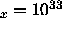ergs s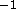in the ROSAT energy band (0.1 to 2.4 keV), and is at a distance of 2 kpc with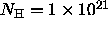cm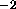(log(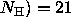). The assumed temperature is equivalent to 0.1 keV. The unabsorbed flux for this source is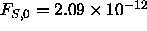ergs cms, or in units of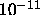the flux is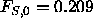. From the Table 11.5or Figure 11.7the appropriate ECF is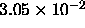, and the expected count rate is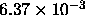counts s. We estimate that a detection of this source at the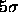level will require an observation time of about 4,000 s.
2. Consider a distant AGN with a power law spectrum of energy slope 0.5 which is at a luminosity distance of 1000 Mpc and is viewed through an absorption column of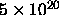cm(log(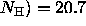). The AGN luminosity in the ROSAT band is L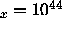ergs s. We want to determine how long an observation is required for a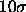detection. The unabsorbed flux is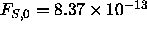ergs cms. The ECF for this spectral model is taken from Table 11.3or Figure 11.5, it is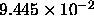, which gives a count rate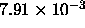counts s. Using the sensitivity formula from § 11.2, we calculate that the observation time needed to detect this source at thelevel is about 12,600 s.
3. What is the conversion factor from counts per second to energy flux for a particular spectrum? For example, a Raymond-Smith Plasma with a temperature corresponding to 1 keV and a column of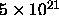cm(log(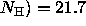). The ECF for this spectral model is taken from Table 11.4or Figure 11.6, it is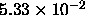. That is, a flux of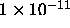ergs cmsin the ROSAT energy band (0.1 to 2.4 keV) will give a count rate ofcounts sin the HRI. Inverting this relationship gives the result that 1 count s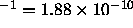ergs cms. In general the conversion factor from counts per second to energy flux is given by: 1 count s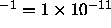/ECF ergs cms.Next: Spatial Resolution Up: Expected Count Rates Previous: Expected Count Rates

Michael Arida
Tue Jun 11 16:18:41 EDT 1996